Wavelet-based multivariate Fourier spectral estimation

Standard nonparametric Fourier spectral matrix estimation

In multivariate time series analysis, the second-order behavior of a multivariate time series is studied by means of the autocovariance matrices in the time domain, or the Fourier spectral density matrix in the frequency domain. A nondegenerate Fourier spectrum is necessarily a curve (or surface) of Hermitian positive definite (HPD) matrices, and one generally constrains a spectral estimator to preserve this property. This in order to ensure interpretability of the estimator as a covariance or spectral density matrix, but also to avoid computational issues in e.g., simulation or bootstrapping.

The package pdSpecEst contains several useful functions to generate vector-valued time series observations and perform standard nonparametric Fourier spectral matrix estimation. First, the function rExamples1D() generates stationary vector-valued time series observations based on a Cramér representation in terms of the transfer function of several example target spectral matrices ((Brillinger 1981)). The orthogonal increment process is generated by means of independent complex normal random variates. Another function that can be used to generate stationary vector-valued time series observations is rARMA(), which generates observations from a vARMA (vector autoregressive-moving-average) process.

library(pdSpecEst)
## Generate 3-dim. time series with 'bumps' spectrum
set.seed(123)
n <- 2^10
bumps <- rExamples1D(n, example = "bumps", return.ts = T, noise = "periodogram")
str(bumps, digits.d = 1)
#> List of 3
#>  $f : cplx [1:3, 1:3, 1:1024] 9e+00+0e+00i 0e+00+1e-14i -8e-15+1e-14i ... #>$ P : cplx [1:3, 1:3, 1:1024] 10+0i -7+4i -7+2i ...
#>  $ts: cplx [1:2048, 1:3] -9+3i 11+15i 0-19i ... If we set return.ts = T, the function rExamples1D() outputs a list with three components: (i) the $$(d \times d)$$-dimensional target HPD spectral matrix f; (ii) a multitaper HPD periodogram of the generated time series, by default calculated with $$B = d$$ DPSS (discrete prolate spheroidal sequence) taper functions; and (iii) the generated time series observations, with the $$d$$ columns corresponding to the components of the time series. Figure 1 displays the true generating spectrum and a noisy multitaper HPD spectral estimator, with $$B = 3$$ DPSS tapers and time-bandwidth parameter $$n_w = 3$$, based on a generated time series of length $$T = 1024$$. In general, to compute a raw or smoothed periodogram of a stationary vector-valued time series, one can use the function pdPgram(). By specifying the argument $$B$$, the function directly computes a multitaper spectral estimator, by default using DPSS tapering functions, with time-bandwidth parameter $$n_w = 3$$. ## Multitaper spectral estimator with pdPgram f.hat <- pdPgram(bumps$ts, B = 75); str(f.hat, digits.d = 1)
#> List of 2
#>  $freq: num [1:1024] 0 0.003 0.006 0.009 0.012 ... #>$ P   : cplx [1:3, 1:3, 1:1024] 14+0i 5+1i -6+0i ...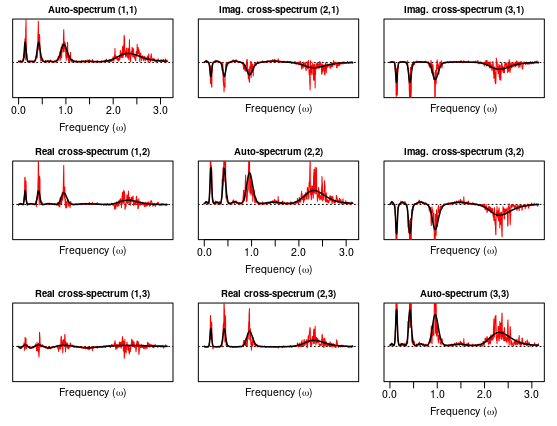Figure 1: Generating HPD spectral matrix bumps$f in black and noisy mulitaper HPD periodogram bumps$P in red. The diagonal components have zero imaginary part and the missing off-diagonal components are uniquely determined by complex conjugation of the displayed off-diagonal components.

Figure 2 displays the multitaper HPD spectral estimator based on the same generated time series of length $$T = 1024$$ using $$B = 75$$ DPSS tapers and time-bandwidth parameter $$n_w = 3$$. Observe that a standard multitaper spectral estimator has difficulties adapting to the varying degrees of smoothness in the target spectrum as the estimation procedure is based on a single global smoothness parameter $$B$$, i.e., the number of tapers.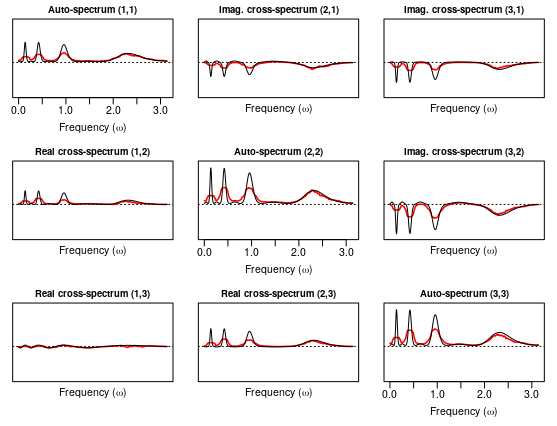Figure 2: Generating HPD spectral matrix bumps$f in black and mulitaper HPD spectral estimator f.hat$P in red. The diagonal components have zero imaginary part and the missing off-diagonal components are uniquely determined by complex conjugation of the displayed off-diagonal components.

More flexible spectral matrix estimation

Although standard nonparametric spectral estimation methods, such as multitapering, are suitable to estimate smooth Fourier spectra with globally smooth component curves across frequency in each individual matrix component, they are not able to capture localized features, such as local peaks in the spectral matrix at certain frequencies or frequency bands, or varying degrees of smoothness across components of the spectral matrix. (Chau and Sachs 2019) develops intrinsic wavelet transforms for curves or surfaces in the space of HPD matrices, with applications to denoising, dimension reduction or compression, and more. The intrinsic wavelet transforms are computationally fast and denoising through nonlinear wavelet shrinkage captures localized features, such as peaks or throughs, in the matrix-valued curves or surfaces, always guaranteeing an estimate in the space of HPD matrices. Moreover, and in contrast to existing approaches, wavelet-based spectral estimation in the space of HPD matrices equipped with a specific invariant Riemannian metric is equivariant under a change or basis (i.e., change of coordinate system) of the given time series. For more details, see (Chau and Sachs 2019) or (Chau 2018).

Wavelet-based spectral matrix estimation with pdSpecEst1D()

In this section, we demonstrate how to use the pdSpecEst package to denoise curves of HPD matrices corrupted by noise via linear or nonlinear thresholding of the intrinsic wavelet coefficients. In particular, we consider the application to spectral matrix estimation of sta- tionary time series through wavelet-based denoising of HPD periodogram matrices. Consider again the generated time series bumps$ts with generating spectral matrix bumps$f and noisy HPD periodogram matrix bumps$P as displayed in Figure 1. The function WavTransf1D() transforms the generating spectral matrix or the noisy HPD periodograms (i.e., curves of HPD matrices) to the intrinsic AI (average-interpolation) wavelet domain. ## Forward intrinsic AI wavelet transform wt.f <- WavTransf1D(bumps$f, periodic = T)
wt.per <- WavTransf1D(bumps$P, periodic = T) By default, the order of the intrinsic AI subdivision scheme is order = 5, and the space of HPD matrices is equipped with: 1. the affine-invariant Riemannian metric as detailed in e.g., (Bhatia 2009)[Chapter 6] or (Pennec, Fillard, and Ayache 2006), i.e., metric = "Riemannian". Instead, the metric can also be specified to be: 1. the log-Euclidean metric, the Euclidean inner product between matrix logarithms (metric = "logEuclidean"); 2. the Cholesky metric, the Euclidean inner product between Cholesky decompositions (metric = "Cholesky"); 3. the Euclidean metric (metric = "Euclidean"); 4. the root-Euclidean metric, the Euclidean inner product between Hermitian matrix square roots (metric = "rootEuclidean"). The default choice of metric (affine-invariant Riemannian) satisfies several useful properties not shared by the other metrics, see (Chau and Sachs 2019) or (Chau 2018) for more details. Note that this comes at the cost of increased computation time in comparison to one of the other metrics, (due to the frequent use of matrix square roots, logarithms and exponentials). If the average-interpolation order satisfies order <= 9, the predicted midpoints in the intrinsic AI subdivision scheme are calculated efficiently by a weighted intrinsic average with pre-determined filter weights. If order > 9, the midpoint prediction is computationally more expensive as it relies on intrinsic polynomial interpolation via Neville’s algorithm with the function pdNeville(). In Figures 3 and 4 below, we plot the Frobenius norms of the matrix-valued whitened AI wavelet coefficients of the true spectral matrix bumps$f and the noisy HPD periodograms bumps$P across scales $$1 \leq j \leq J$$ and locations $$0 \leq k \leq 2^{j-1} - 1$$, with maximum wavelet scale $$J= \log_2(n) = 10$$.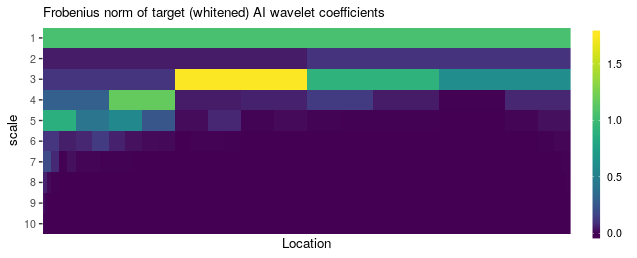Figure 3: Frobenius norms of the whitened AI wavelet coefficients of the generating spectral matrix bumps$f based on an intrinsic AI wavelet transform of order $$N = 5$$, under the default affine-invariant metric, obtained with WavTransf1D().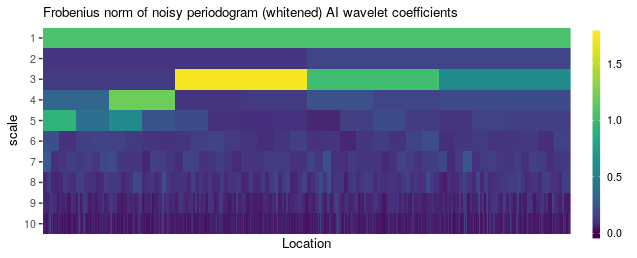Figure 4: Frobenius norms of the whitened AI wavelet coefficients of the noisy HPD periodogram bumps$P based on an intrinsic AI wavelet transform of order $$N = 5$$, under the default affine-invariant metric, obtained with WavTransf1D(). Given as input the curve of noisy HPD periodograms bumps$P, the function pdSpecEst1D() computes a HPD wavelet-denoised spectral matrix estimator by applying the following steps:

1. Application of a forward intrinsic AI wavelet transform, with WavTransf1D(),
2. (Tree-structured) thresholding of the wavelet coefficients, with pdCART(),
3. Application of an inverse intrinsic AI wavelet transform, with InvWavTransf1D().

The complete estimation procedure is described in more detail in (Chau and Sachs 2019) or Chapter 3 of (Chau 2018). By default, the function applies the intrinsic wavelet transform based on the affine-invariant Riemannian metric, and corresponding (asymptotic) bias-correction as in Chapter 3 of (Chau 2018).

f.hat <- pdSpecEst1D(bumps$P, return.D = "D.white"); str(f.hat, max.level = 1) #> List of 6 #>$ f           : cplx [1:3, 1:3, 1:1024] 5.97-0i 2.69+6.11i -6.35+2.4i ...
#>  $D :List of 9 #>$ M0          : cplx [1:3, 1:3, 1:5] 11.132-0i -0.335-1.859i 0.964-0.498i ...
#>  $tree.weights:List of 8 #>$ D.raw       :List of 9
#>  $D.white :List of 9 The function outputs a list with several components, among which the most important are the denoised HPD matrix curve f, and the pyramid of thresholded wavelet coefficients D. See the complete package documentation for additional information on the functions used in the intermediate steps and more details on the specifics of the function pdSpecEst1D(). Nonlinear tree-structured wavelet thresholding By default, the noise is removed by tree-structured thresholding of the wavelet coefficients based on the trace of the whitened coefficients through minimization of a complexity penalized residual sum of squares (CPRESS) criterion via the fast tree-pruning algorithm in (Donoho 1997). The penalty or sparsity parameter in the optimization procedure is set equal to alpha times the universal threshold, where the noise standard deviation (homogeneous across scales for independent Wishart matrices) of the traces of the whitened wavelet coefficients is determined via the median absolute deviation (MAD) of the coefficients at the finest wavelet scale. Linear wavelet thresholding It is also possible to perform linear thresholding of wavelet scales using pdSpecEst1D() by setting the argument alpha = 0, (i.e., no nonlinear thresholding), and the argument jmax to the maximum wavelet scale we wish to keep in the intrinsic inverse AI wavelet transform. For instance, if jmax = 5, the wavelet coefficients at scales $$j$$ with $$j \leq 5$$ remain untouched, but all wavelet coefficients at scales $$j > 5$$ will be set to zero. By default, the function pdSpecEst1D() sets the maximum nonzero wavelet scale to $$J-2$$, two scales below the finest wavelet scale $$J$$. Estimation results In Figure 5, we displaye the Frobenius norms of the Hermitian matrix-valued whitened AI wavelet coefficients of the denoised HPD spectral matrix estimator across scale-locations $$(j, k)$$, and in Figure 6, we plot the wavelet-denoised HPD spectral estimator obtained from f.hat$f together with the generating spectral matrix bumps$f in the same fashion as in Figure 2 above. The spectral matrix estimator captures both the smooth spectral matrix behavior in the second half of the frequency domain and the localized peaks in the low-frequency range, while always guaranteeing positive definiteness of the estimator.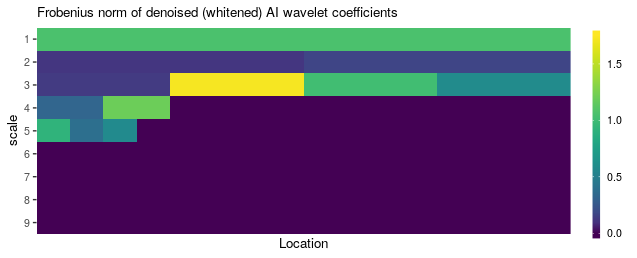Figure 5: Frobenius norms of the nonlinear (tree-structured) thresholded whitened AI wavelet coefficients of the noisy HPD periodograms based on an intrinsic AI wavelet transform of order $$N = 5$$, under the default affine-invariant metric.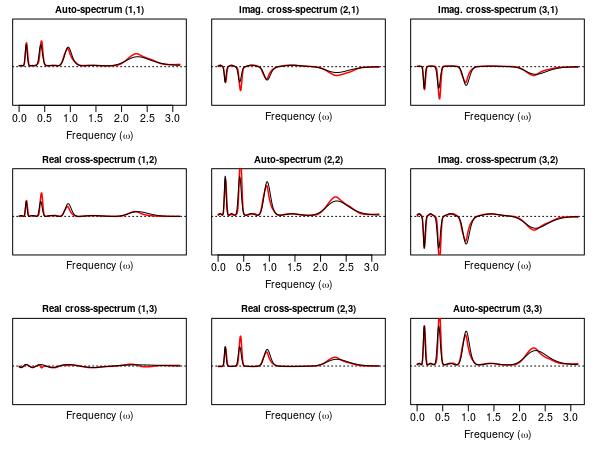Figure 6: Generating spectrum bumps$f in black and wavelet-smoothed HPD spectral estimator f.hat$f in red. The diagonal components have zero imaginary part and the missing off-diagonal components are uniquely determined by complex conjugation of the displayed off-diagonal components. Wavelet-based spectral matrix clustering with pdSpecClust1D() The intrinsic AI wavelet transforms can also be used for fast clustering of multivariate spectral matrices based on their sparse representations in the wavelet domain. The function pdSpecClust1D() performs clustering of HPD spectral matrices corrupted by noise (e.g., multitaper HPD periodograms) by combining wavelet thresholding and fuzzy clustering in the intrinsic wavelet coefficient domain. In particular, the HPD spectral matrices are assigned to a number different clusters in a probabilistic fashion according to the following steps: 1. Transform a collection of noisy HPD spectral matrices to the intrinsic wavelet domain and denoise the HPD matrix curves by (tree-structured) thresholding of wavelet coefficients with pdSpecEst1D(). 2. Apply an intrinsic fuzzy c-means algorithm to the coarsest midpoints at scale j = 0 across subjects, see e.g., (Bezdek 1981). Note that the distance function in the intrinsic c-means algorithm relies on the chosen metric on the space of HPD matrices. 3. Taking into account the fuzzy cluster assignments in the previous step, apply a weighted fuzzy c-means algorithm, based on the Euclidean distance function, to the nonzero thresholded wavelet coefficients across subjects from scale j = 1 up to j = jmax. The tuning parameter tau controls the weight given to the cluster assignments obtained in the first step of the clustering algorithm. Example of HPD spectral matrix clustering As an illustrating example, we simulate stationary two-dimensional time series data for ten different subjects from two vARMA(2,2) (vector-autoregressive-moving-average) processes with slightly different coefficient matrices, and thus non-equal spectral matrices, using the function rARMA(). Here, the first group of five subjects is simulated from a first vARMA-process and the second group of five subjects is simulated from a second slightly different vARMA-process. We use pdSpecClust1D() to assign the different subjects to K = 2 clusters in a probabilistic fashion. Note that the true clusters are formed by the first group of five subjects and the last group of five subjects. Wavelet-based time-varying spectral matrix estimation with pdSpecEst2D() Instead of denoising curves of HPD matrices, the pdSpecEst package can also be used to denoise surfaces of HPD matrices corrupted by noise. This is done through linear or nonlinear shrinkage of wavelet coefficients obtained by means of an intrinsic 2D AI wavelet transform as explained in Chapter 5 of (Chau 2018). In particular, we focus on denoising of HPD surfaces of random Wishart matrices, with in mind the application to time-varying spectral matrix estimation based on wavelet denoising of time-varying periodogram matrices. The primary tool to perform intrinsic wavelet denoising of noisy surfaces of HPD matrices is the function pdSpecEst2D(). The functions below are highly similar to the previously demonstrated functions, where the suffix -1D in the context of curves of HPD matrices is replaced by the suffix -2D in the context of surfaces of HPD matrices. Simulate noisy HPD surfaces with rExamples2D() First, to generate a noisy surface of size $$n_1 \times n_2$$ in the space of $$(d \times d)$$-dimensional HPD matrices $$\mathbb{P}_{d \times d}$$, we use the function rExamples2D(), with the argument noise = 'wishart', which generates HPD matrix observations from a discretized intrinsic signal plus i.i.d. noise model with respect to the Riemannian metric: $P_{ij} = f_{ij}^{1/2} E_{ij} f_{ij}^{1/2} \in \mathbb{P}_{d \times d}, \quad 1 \leq i \leq n_1, 1 \leq i \leq n_2,$ with target surface $$(f_{ij})_{ij} \in \mathbb{P}_{d \times d}$$ and noise $$(E_{ij})_{ij} \in \mathbb{P}_{d \times d}$$ generated from a complex Wishart distribution $$W_d^C(B, \text{Id}/B)$$, with $$B$$ degrees of freedom and Euclidean mean equal to the $$(d \times d)$$-dimensional identity matrix $$\text{Id}$$, such that the Euclidean mean of $$P_{ij}$$ equals $$f_{ij}$$. Informally, such random matrix behavior corresponds to the asymptotic behavior of localized or segmented HPD periodogram observations (e.g., obtained with pdPgram2D()) of a locally stationary time series, with generating time-varying spectral matrix equal to the target surface $$(f_{ij})_{ij}$$, see e.g., Example 5.4.1 in (Chau 2018). Figures 7 and 8 display the matrix-logarithms $$\text{Log}(f_{ij}) \in \mathbb{H}_{d \times d}$$ of the (true) HPD target surface and the matrix-logarithms of the generated HPD matrix observations $$\text{Log}(P_{ij}) \in \mathbb{H}_{d \times d}$$ corresponding to the target surface corrupted by Wishart noise, where $$\mathbb{H}_{d \times d}$$ denotes the space of $$(d \times d)$$-dimensional Hermitian matrices.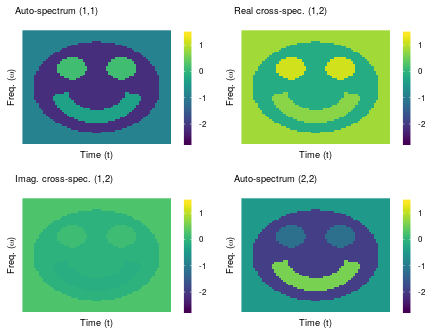Figure 7: Matrix-logarithms of the target surface of HPD matrices smiley$f in time and frequency. The diagonal components have zero imaginary part and the missing off-diagonal matrix components are uniquely determined by complex conjugation of the displayed off-diagonal components.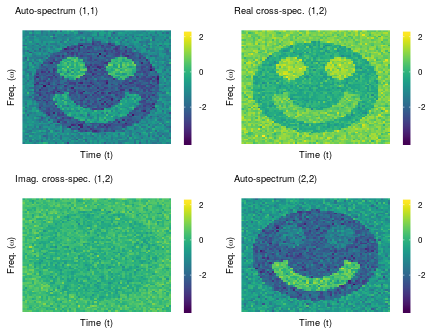Figure 8: Matrix-logarithms of generated noisy surface of HPD matrices smiley$P in time and frequency. The diagonal components have zero imaginary part and the missing off-diagonal matrix components are uniquely determined by complex conjugation of the displayed off-diagonal components. The function pdSpecEst2D() computes a wavelet-denoised HPD surface estimator by applying the following steps to an initial noisy HPD surface (obtained with e.g., pdPgram2D()): 1. Application of a forward intrinsic 2D AI wavelet transform, with WavTransf2D(), 2. (Tree-structured) thresholding of the wavelet coefficients, with pdCART(), 3. Application of an inverse intrinsic 2D AI wavelet transform, with InvWavTransf2D(). For more details on the functions used in the intermediate steps, we refer to the complete package documentation. Currently, the intrinsic forward and inverse 2D AI wavelet transforms act only on dyadic grids using a natural dyadic refinement pyramid as explained in Chapter 5 of (Chau 2018). By default, the marginal average-interpolation orders of the forward and inverse 2D wavelet transform are set to order = c(3, 3), and the predicted midpoints in the 2D AI subdivision scheme are calculated efficiently by means of local intrinsic weighted averages using pre-determined filter weights. As in the 1D forward and inverse AI wavelet transforms above, by default, the intrinsic wavelet transform is computed with respect to the affine-invariant Riemannian metric, i.e., metric = "Riemannian", but this can also be one of: "logEuclidean", "Cholesky", "rootEuclidean" or "Euclidean". The function pdSpecEst2D() outputs a list with several components, among which the most important are the denoised HPD surface f at the given rectangular observation grid, and the pyramid of thresholded wavelet coefficients D. See the function documentation for more information about its specific arguments and outputs. Nonlinear and linear wavelet thresholding By default, shrinkage of the wavelet coefficients in the function pdSpecEst2D() is carried out through nonlinear tree-structured thresholding of entire matrix-valued wavelet coefficients based on the trace of the whitened coefficients. This is done in the same way as before through minimization of a CPRESS criterion via a fast tree-pruning algorithm as in (Donoho 1997). For thresholding of 2D wavelet coefficients on a non-square time-frequency grid, there is a discrepancy between the constant noise variance of the traces of the whitened coefficients at the first $$|J_1 - J_2|$$ coarse scales and the remaining finer scales, where $$J_1 = \log_2(n_1)$$ and $$J_2 = \log_2(n_2)$$ with $$n_1$$ and $$n_2$$ the (dyadic) number of observations in each marginal direction of the $$(n_1 \times n_2)$$-dimensional observation grid. To correct for this discrepancy, the variances are normalized to a unit noise variance across wavelet scales via the semiparametric method described in Chapter 5 of (Chau 2018). Note that if the observation grid is square, i.e., $$n_1 = n_2$$, the variances of the traces of the whitened coefficients are homogeneous across all wavelet scales as in the 1D context described above. The penalty parameter $$\lambda$$ in the CPRESS criterion is set equal to the universal threshold rescaled by a factor $$\alpha$$, i.e., $$\lambda = \alpha \sqrt{2 \log(N)}$$, where $$N$$ is the total number of pooled coefficients, and by default the rescaling factor $$\alpha$$ is equal to 1, (alpha = 1), such that $$\lambda$$ equals the universal threshold. Analogous to the function pdSpecEst1D(), linear thresholding of wavelet scales is performed by specifying the argument alpha = 0, (i.e., zero nonlinear threshold), and setting the argument jmax to the maximum nonzero wavelet scale to be kept in the inverse wavelet transform. By default, the function pdSpecEst2D() sets the maximum nonzero wavelet scale jmax to $$J-2$$, two scales below the finest wavelet scale $$J$$. Figure 9 displays the matrix-logarithms of the nonlinear wavelet-thresholded HPD surface f.hat$f in the same fashion as plotted in Figures 7 and 8 above.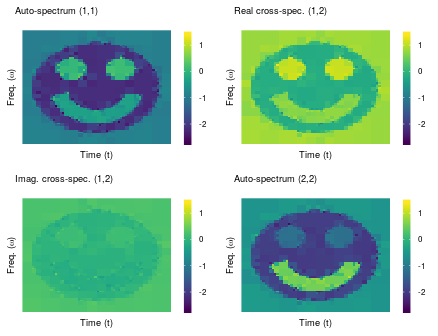Figure 9: Matrix-logarithms of denoised surface of HPD matrices f.hat\$f in time and frequency. The diagonal components have zero imaginary part and the missing off-diagonal matrix components are uniquely determined by complex conjugation of the displayed off-diagonal components.